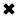# Active Power#### Active Power: Average power, real power, or true power

Active power is the power which is actually consumed or utilized in an AC Circuit is called True power or Active Power or real power. It is measured in kilowatt (kW) or MW. It is the actual outcomes of the electrical system which runs the electric circuits or load.

Active power, P, is also commonly referred to as the average power, real power, or true power. It represents useful power expended by loads to perform real work, that is, to convert electric energy to other forms of energy. Real work performed by an incandescent light bulb is to convert electric energy into light and heat. In electric power, real work is performed for the portion of the current ihat is in phase with the voltage. No real work will result, from the portion where the current is not in phase with the voltage. Real work performed by an incandescent light bulb is to convert electric energy into light and heat. In electric power, real work is performed for the portion of the current ihat is in phase with the voltage. No real work will result, from the portion where the current is not in phase with the voltage.  The active power is the rate at which energy is expended, dissipated or consumed by the load, and is measured in units of watts (W). P can be computed by averaging the product of the instantaneous voltage and current, that is,Equation 1

The above active power equation is valid for both sinusoidal and nonsinusoidal conditions. For sinusoidal condition, '1rn, resolves to the familiar form,Equation 2

where O is the phase angle between voltage and current at the fundamental frequency. Equation 2 indicates that the average active power is a function only of the fundamental frequency quantities. In the nonsinusoidal case, the computation of the active power must include contribution from all harmonic components, thus it is the sum of active power at each harmonic. Furthermore, because the voltage distortion is generally very low on power systems (less than 5%), Equation 2 is a good approximation regardless of how distorted the current is. This approximation cannot be applied when computing the apparent and reactive power. These two quantities are greatly influenced by the distortion. The apparent power, S, is a measure of the potential impact of the load on the thermal capability of the system. It is proportional to the rms of the distorted current and its computation is straightforward, although slightly more complicated than the sinusoidal case. Also, many current probes can now directly report the true rms value of a distorted waveform.

##### Basic Electricity Handbook, Vol. 1• FULLY ILLUSTRATED
• GREAT FOR SCHOOL PROJECTS
• GREAT PRICE: \$5.99
• ...

This 100+ page e-book is a great guide for those who have a basic interest in the field of electricity. This well-illustrated e-book, coupled with some basic knowledge of electricity, will give you a broad theoretical background in this fundamental subject.

CONTENTS
• FUNDAMENTALS OF ELECTRICITY
• HOW DOES ELECTRICITY WORK?
• HOW IS ELECTRICITY GENERATED?
• WHERE DOES ELECTRICITY COME FROM?
• BASIC HOME WIRING
• ELECTRICITY FOR STUDENTS
• ENERGY SAVING TIPS

##### TRAINING EF COURSES

Content Community Connection
Top